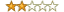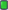Thread: hashcat and HMAC-SHA256 ... View Single Post
#4
 chantsFamilyJoin Date: Jul 2016 Posts: 476 Rept. Given: 2 Rept. Rcvd 33 Times in 19 Posts Thanks Given: 404 Thanks Rcvd at 751 Times in 345 PostsFirst off the relevant file for this change (assuming you are using the 1450 variant which it sure sounds like you are) - size independent files:
Quote:
 https://github.com/hashcat/hashcat/blob/master/OpenCL/inc_hash_sha256.h https://github.com/hashcat/hashcat/blob/master/OpenCL/inc_hash_sha256.cl https://github.com/hashcat/hashcat/blob/master/OpenCL/m01450_a0-pure.cl https://github.com/hashcat/hashcat/blob/master/OpenCL/inc_rp.cl
This chain led me to:
Quote:
 https://github.com/hashcat/hashcat/blob/master/OpenCL/inc_types.h
Code:
```typedef struct pw
{
u32 i;
u32 pw_len;
} pw_t;```
So sizeof(u32)*64 = 256...change to sizeof(u32)*2048=8192. I am assuming you made exactly this change and recompiled e.g. u32 i;

I don't know why it would crash - perhaps you can use a debugger and give the source code line upon which it crashes. I am assuming you are using OpenCL and not GPU though I would have imagined they would share definitional source.

It does call sha256_hmac_init_swap which has special handling above 64 size - the truncation to 8 bytes looks strange and maybe this is untested given the buffer limitation? It in turn calls sha256_update_swap which looks like it handles any size. Based on the spec:
Quote:
 https://en.wikipedia.org/wiki/HMAC
it would seem this is correct behavior as 256 bit output is 32 bytes.

It would be nice to make a PR for this but because of the optimization sensitive nature of this project, more thinking about exactly how to do that is needed - maintain optimization while allowing the buffer size to change without annoying recompilations.

Oh and finally this is likely the issue - code needs to be generalized in:
Quote:
 https://github.com/hashcat/hashcat/blob/master/src/modules/module_01450.c
First change:
Code:
```  token.len_min = SALT_MIN;
token.len_max = SALT_MAX;```
Next change:
Code:
```  digest = hex_to_u32 (hash_pos +  0);
digest = hex_to_u32 (hash_pos +  8);
digest = hex_to_u32 (hash_pos + 16);
digest = hex_to_u32 (hash_pos + 24);
digest = hex_to_u32 (hash_pos + 32);
digest = hex_to_u32 (hash_pos + 40);
digest = hex_to_u32 (hash_pos + 48);
digest = hex_to_u32 (hash_pos + 56);
digest = byte_swap_32 (digest);
digest = byte_swap_32 (digest);
digest = byte_swap_32 (digest);
digest = byte_swap_32 (digest);
digest = byte_swap_32 (digest);
digest = byte_swap_32 (digest);
digest = byte_swap_32 (digest);
digest = byte_swap_32 (digest);```

Last edited by chants; 08-19-2019 at 08:13.